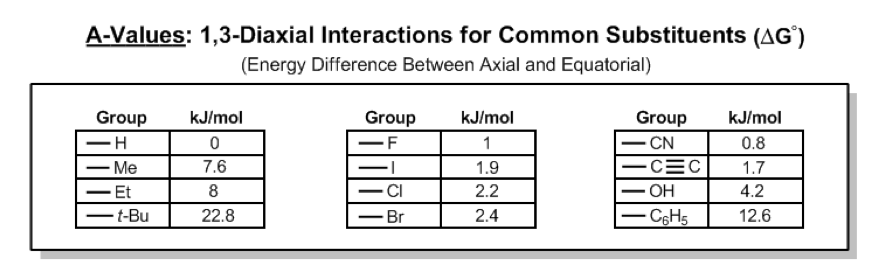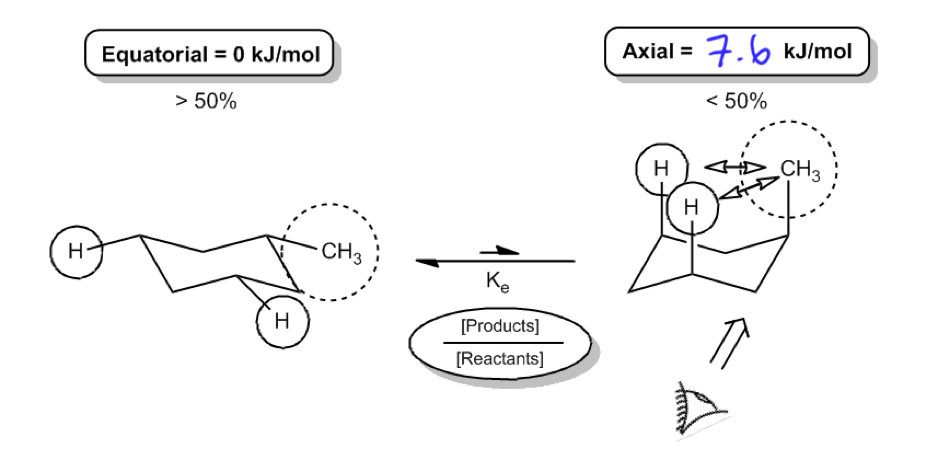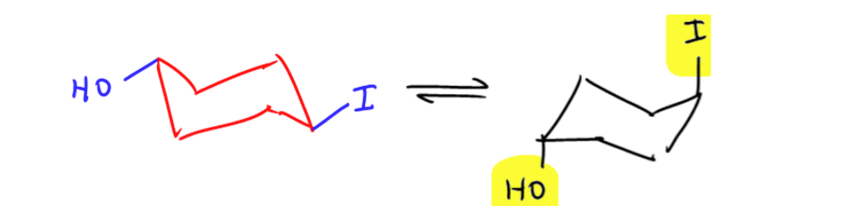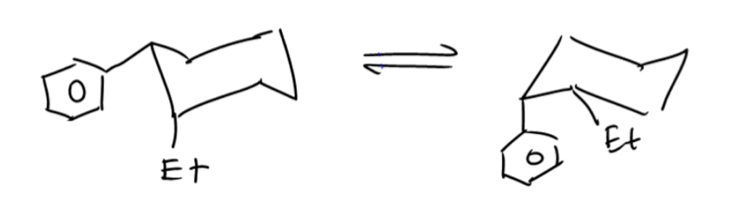Start typing, then use the up and down arrows to select an option from the list.# Organic Chemistry

Learn the toughest concepts covered in Organic Chemistry with step-by-step video tutorials and practice problems by world-class tutors.

4. Alkanes and Cycloalkanes

# Calculating Energy Difference Between Chair Conformations

For most classes all you will need to know how to do is use equatorial preference to predict the most stable chair conformation.

However, sometimes you will be required to use energetics to calculate the exact percentages of each chair in solution. This is a multistep process, so here I’m going to walk you through it from scratch.

## Calculating Flip Energy

First we have to introduce the concept of an A-value, which is simply the energy difference between the equatorial (most stable) and axial (least stable) positions.

1

#### concept

Explaining how A-Values are related to cyclohexane flip energy9m
Play a video:We can use these values to calculate how much energy it is going to take to flip a chair into its least stable form.Note:The above chair flip in the video is slightly off. Remember that the direction of the groups (up vs. down) should not change when going from axial to equatorial or vice versa.

All the math is still correct here, but I should have drawn the groups down instead of up on the second chair.:)

[Refer to the videos below for examples of this]

2
Problem

Calculate the difference in Gibbs free energy between the alternative chair conformations of trans-4-iodo-1-cyclohexanol.3
Problem

Calculate the difference in Gibbs free energy between the alternative chair conformations of cis-2-ethyl-1-phenylcyclohexane.